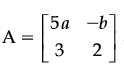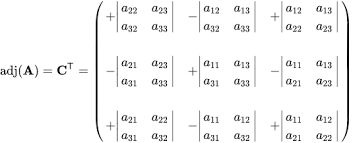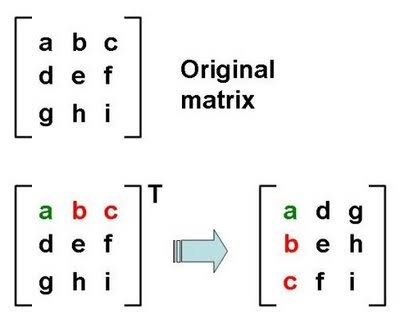#### Ifand A adj A=A AT, then5 a + b is equal to : Option 1) -1 Option 2) 5 Option 3) 4 Option 4) 13As we learnt in

Adjoint of a square matrix -

Transpose of the matrix of co-factors of elements ofis called the adjoint of- whereinTranspose of a Matrix -

The matrix obtained from any given matrix, by interchanging its rows and columns.

- whereinso that 15a - 2b = 0

and 10a + 3b =13Option 1)

-1

This option is incorrect.

Option 2)

5

This option is correct.

Option 3)

4

This option is incorrect.

Option 4)

13

This option is incorrect.

#### divya.saini